## 分布式运动约束下悬臂输液管的参数共振研究 1)

* 湖北文理学院机械工程学院,湖北襄阳 441053

† 华中科技大学力学系,工程结构分析与安全评定湖北省重点实验室,武汉 430074;

## PARAMETRIC RESONANCE OF A CANTILEVERED PIPE CONVEYING FLUID SUBJECTED TO DISTRIBUTED MOTION CONSTRAINTS 1)

Wang Yikun*,2), Wang Lin†,3)

* School of Mechanical Engineering, Hubei University of Arts and Science, Xiangyang 441053, Hubei,China

† Hubei Key Laboratory for Engineering Structural Analysis and Safety Assessment, Department of Mechanics, Huazhong University of Science and Technology, Wuhan 430074, China;

 基金资助: “机电汽车”湖北省优势特色学科群开放基金.  XKQ2018069国家自然科学基金.  11622216

Received: 2018-09-4   Accepted: 2018-11-28   Online: 2019-03-18

2)王乙坤,讲师,主要研究方向:流固耦合动力学,动力学与控制.E-mail:wangyikun18@hbuas.edu.cn

3)王琳,教授,主要研究方向:流固耦合动力学,动力学与控制.E-mail:wanglindds@hust.edu.cnAbstract

Pipes conveying fluid have been widely used in the fields of aerospace, mechanics, marine, hydraulic and nuclear engineering. The stability analysis, dynamic response and safety assessment of fluid-conveying pipes subjected to nonlinear constraints are particularly important for both engineering applications and scientific researches. Although the dynamical behaviors of fluid-conveying pipes subjected to single-point loose constraints have been discussed for four decades, the literature on the dynamics of fluid-conveying pipes subjected to distributed motion constraints is very limited. To obtain a better understanding of the dynamics of a cantilevered pipe conveying fluid subjected to distributed motion constraints, both cubic and modified trilinear spring models are employed in this study to describe the restraining force between the pipe and the motion constraints. This study is also concerned with the parametric resonance when the pipe is excited by an internal pulsating fluid. Firstly, the modified nonlinear equation of the pipe system was discretized via Galerkin's approach and solved using a fourth-order Runge-Kutta method. Via the Floquet theory, the nonlinear equation of motion was simplified to a linear one to calculate the parametric resonance regions versus the pulsating amplitude and frequency. Two representative values of mean flow velocity were employed to calculate the parametric resonance regions. Both the two values of mean flow velocity are assumed to be lower than the critical velocity for the cantilevered pipe system. Then, considering the geometric nonlinearity, the nonlinear dynamic responses focusing on the effect of external nonlinear restraining forces generated by the distributed motion constraints are discussed in detail. Results show that the stability regions of the nonlinear system agree well with that predicted by analyzing the linearized system. It is found that the distributed motion constraints would mainly affect the displacement amplitudes. Various oscillation types may arise when the pulsating frequency of the flow velocity is varied. Several bifurcation diagrams show that, however, a significant difference can be observed between the routes to chaos for the two constraint models, i.e., the pipe with a trilinear spring model can exhibit chaotic oscillations more easily than that with a cubic spring model.

Keywords： pipe conveying fluid ; pulsating flow ; motion constraint ; nonlinear vibration ; parametric resonance

Wang Yikun, Wang Lin. PARAMETRIC RESONANCE OF A CANTILEVERED PIPE CONVEYING FLUID SUBJECTED TO DISTRIBUTED MOTION CONSTRAINTS 1). Chinese Journal of Theoretical and Applied Mechanics[J], 2019, 51(2): 558-568 DOI:10.6052/0459-1879-18-295

## 1 理论模型

### 图1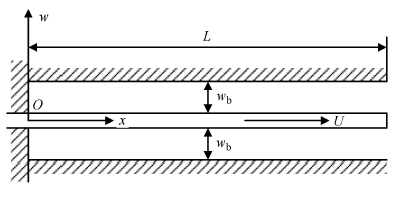Fig.1   Schematic of a cantilever pipe conveying fluid subjected to distributed motion constraints

$\alpha \dot{\eta }"" + {\eta }"" + 2u\sqrt \beta {\dot {\eta }}' + {\eta }"\left[ {u^2 + \left( {\dot {u}\sqrt \beta-\gamma } \right)\left( {1-\xi } \right)} \right] + \gamma {\eta }' + \ddot {\eta } + 2u\sqrt \beta \dot {{\eta }'}{\eta }'^2 + \frac{1}{2}\left( {\dot {u}\sqrt \beta-\gamma } \right){\eta }'^3 + 3{\eta }'{\eta }"{\eta }"' + {\eta }'^2{\eta }"\left[ {u^2 + \frac{3}{2}\left( {\dot {u}\sqrt \beta-\gamma } \right)\left( {1-\xi } \right)} \right] + {\eta }"^3 + {\eta }'∫_{0}^\xi {\left\{ {{\dot {\eta }}'^2-2u\sqrt \beta {\eta }'{\dot {\eta }}" + {\eta }"{{{{\eta }'}'}'}'-} \right.} {\eta }'{\eta }"' \cdot \left. {\left[ {u^2 + \left( {\dot {u}\sqrt \beta-\gamma } \right)\left( {1-\xi } \right)} \right]} \right\} d \xi-{\eta }"\int_\xi ^1 {\int_0^\xi {\left\{ {{\dot {\eta }}'^2-2u\sqrt \beta {\eta }'{\dot {\eta }}" + {\eta }"{\eta }""-{\eta }'{\eta }"'\left[ {u^2 + \left( {\dot {u}\sqrt \beta-\gamma } \right)\left( {1-\xi } \right)} \right]} \right\}d \xi } d \xi }-{\eta }"\int_\xi ^1 {\left[ {\left( {\dot {u}\sqrt \beta-\gamma } \right){\eta }'^2 + 2u\sqrt \beta {\eta }'{\dot {\eta }}' + u^2{\eta }'{\eta }" + {\eta }"{\eta }"'} \right]d \xi } + f\left( \eta \right) = 0$

$$\xi = \frac{x}{L} , \quad \eta = \frac{w}{L} ,\quad \tau = \sqrt {\frac{EI}{m + M}} \frac{t}{L^2} \\ u = \sqrt {\frac{M}{EI}} LU , \quad \alpha = \sqrt {\frac{EI}{m + M}} \frac{E^\ast }{L^2} \\ \beta = \frac{M}{m + M} , \quad \gamma = \frac{m + M}{EI}L^3g$$

(1)立方非线性模型

$$f\left( \eta \right) = \kappa _{\rm c} \eta ^3$$

(2)修正三线性模型

$$f\left( \eta \right) = \kappa _{\rm t} \left[ {\eta-\frac{1}{2}\left( {\left| {\eta + d} \right|-\left| {\eta-d} \right|} \right)} \right]^3$$

$$\eta \left( {\xi ,\tau } \right) = \sum\limits_{j = 1}^N {\varphi _j \left( \xi \right)q_j \left( \tau \right)}$$

$$M \ddot {q} + C \dot{q} + K \pmb {q} + g_1 (q, \dot{q}) + g_2 (q, \dot{q}) + g_3 (q, \dot{q}) + f(q) = 0$$

$$\left. {\pmb g}_{1i}({\pmb q},\dot{\pmb q}) = \alpha _{ ijkl } \cdot {\pmb q}_{j} {\pmb q}_{k} {\pmb q}_{l} \\ {\pmb g}_{2i}({\pmb q},\dot{\pmb q}) = \beta _{ ijkl } \cdot {\pmb q}_{j} {\pmb q}_{k} \dot{\pmb q}_{l} \\ {\pmb g}_{3i}({\pmb q},\dot{\pmb q}) = \gamma_{ ijkl } \cdot {\pmb q}_{j} \dot{\pmb q}_{k} \dot{\pmb q}_{l} \right\}$$

$$\dot{\pmb z} = {\pmb A}z + {\pmb F} + {\pmb G}$$

$$\left. {\pmb A} = \left[ \begin{array}{cc} {\bf 0} & {\pmb I}-{\pmb K} &-{\pmb C} \end{array} \right] , \quad {\pmb F} = \left[ \begin{array}{c} {\bf 0}-{\pmb f} \left( {\pmb q} \right) \end{array} \right] {\pmb G }= \left[ \begin{array}{c} {\bf 0}-{\pmb g}_1 \left( {{\pmb q}, \dot{\pmb q}} \right)-{\pmb g}_2 \left( {{\pmb q},\dot{\pmb q}} \right)-{\pmb g}_3 \left( {{\pmb q},\dot {\pmb q}} \right) \end{array} \right] \right\}$$

$$\dot{\pmb z} = {\pmb A} \left({ \tau }\right) {\pmb z}$$

$${\pmb Z}\left( {{\rm T} + \tau } \right) = \bar{\pmb A}\left( \tau \right) {\pmb Z}\left( \tau \right)$$

$\left[ {{\pmb Z}_1 \left( \tau \right),{\pmb Z}_2 \left( \tau \right), \cdots ,{\pmb Z}_{2n} \left( \tau \right)} \right] = \left[ \begin{array}{cccc} 1 & & & 0 \\ & 1 & & \\ & & \ddots & \\ 0 & & & 1 \end{array} \right]_{2n\times 2n}$

## 2 线性系统的参数共振稳定性

Table 1  The first four dimensionless natural frequencies of the pipe conveying fluid under two flow velocities

NaturalFirst order Second order Third order Fourth order
frequency^1^2以3^4
U0 = 66.2717.6452.00104.60
U0 = 72.7816.8446.1498.47

### 图2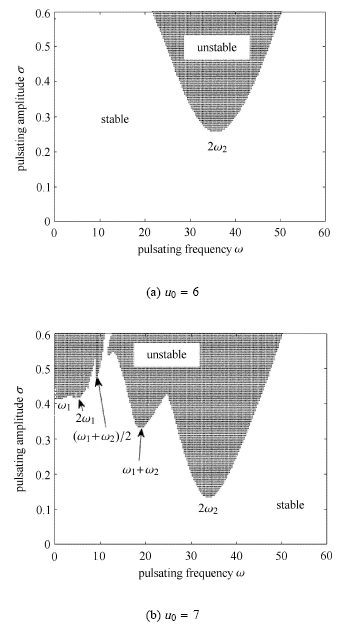Fig.2   Parametric resonance regions of the pipe system under two mean flow velocities

## 3 非线性系统的参数共振响应

### 图3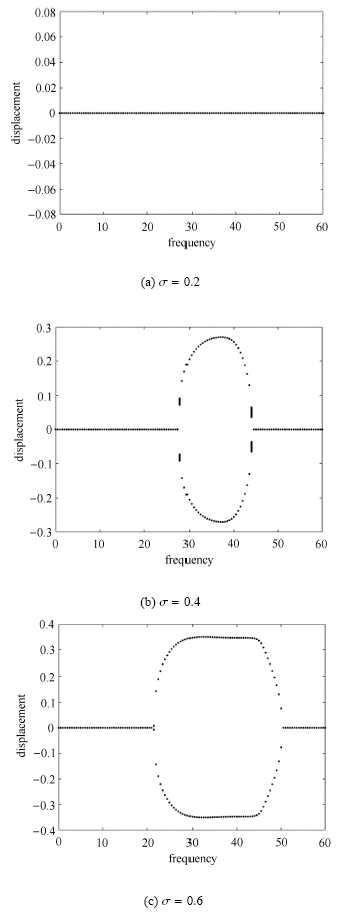Fig.3   Bifurcation diagrams for the tip displacement under various pulsating amplitudes without motion constraints ($u_0 = 6)$

### 图4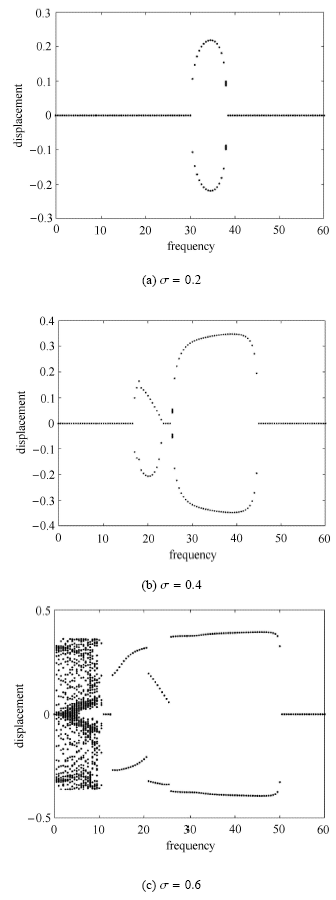Fig.4   Bifurcation diagrams for the tip displacement under various pulsating amplitudes without motion constraints ($u_0 = 7)$

### 图5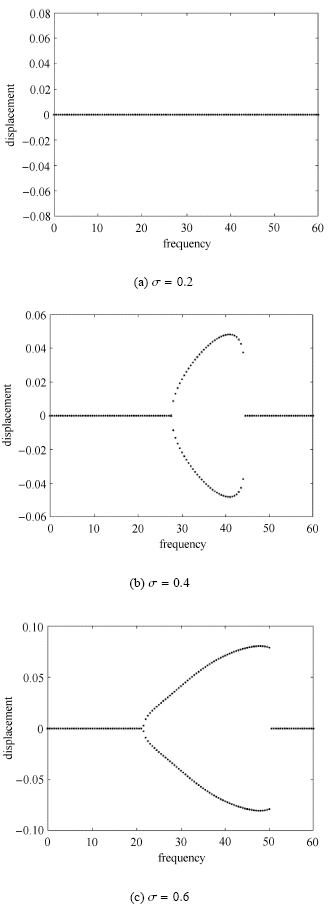Fig.5   Bifurcation diagrams for the tip displacement under various pulsating amplitudes with cubic motion constraints ($u_0 = 6)$

### 图6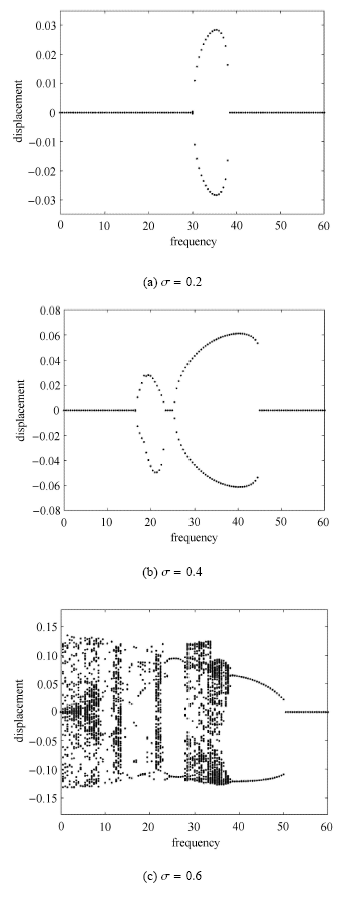Fig. 6   Bifurcation diagrams for the tip displacement under various pulsating amplitudes with cubic motion constraints ($u_0 = 7$)

### 图7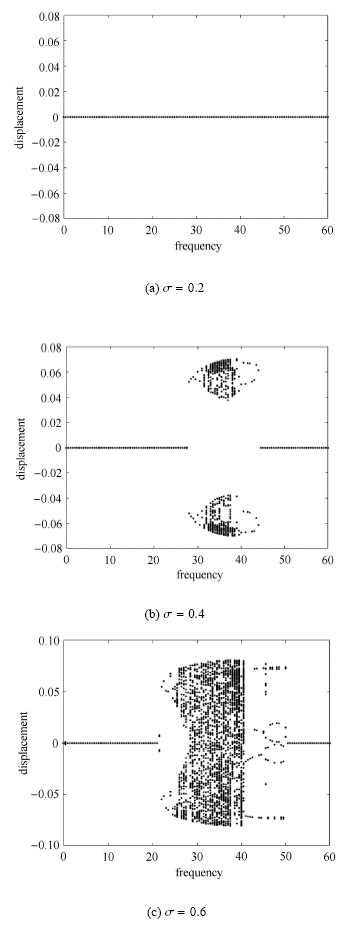Fig.7   Bifurcation diagrams for the tip displacement under various pulsating amplitudes with trilinear motion constraints ($u_0 = 6)$

### 图8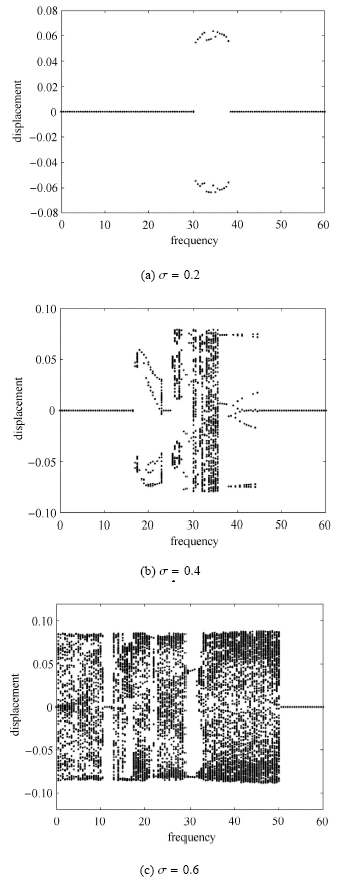Fig.8   Bifurcation diagrams for the tip displacement under various pulsating amplitudes with trilinear motion constraints ($u_0 = 7)$

### 图9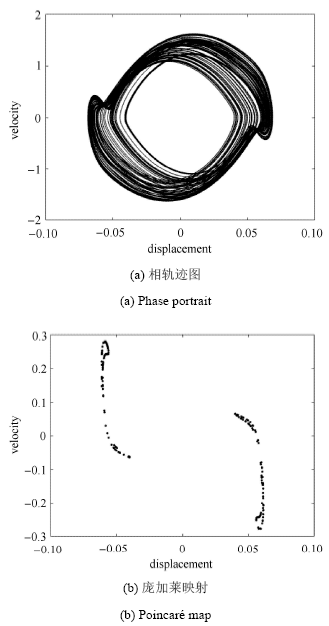Fig.9   Phase portrait and Poincaré map for the pipe system with trilinear motion constraints ($u_0 = 6$,$\sigma = 0.4$,$\omega = 35$)

## 4 结 论

The authors have declared that no competing interests exist.

## 参考文献 原文顺序 文献年度倒序 文中引用次数倒序 被引期刊影响因子

Païdoussis MP. Fluid-Structure Interactions: Slender Structures and Axial Flow. Volume 1. London: Academic Press, 1998

Païdoussis MP. Fluid-Structure Interactions: Slender Structures and Axial Flow. Volume 2. London: Academic Press, 2004

Païdoussis MP, Semler C .

Non-linear dynamics of a fluid-conveying cantilevered pipe with a small mass attached at the free end

International Journal of Non-Linear Mechanics, 1998,33(1):15-32

URL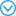( Jin Jiduo, Zou Guangsheng, Zhang Yufei .

Bifurcations and chaotic motions of a cantilevered pipe conveying fluid

Chinese Journal of Theoretical and Applied Mechanics, 2002,34(6):863-873 (in Chinese))

URLNikolić M, Rajković M .

Bifurcations in nonlinear models of fluid-conveying pipes supported at both ends

Journal of Fluids and Structures, 2006,22(2):173-195

URLStationary bifurcations in several nonlinear models of fluid conveying pipes fixed at both ends are analyzed with the use of Lyapunov–Schmidt reduction and singularity theory. Influence of the gravitational force, curvature and vertical elastic support on various properties of bifurcating solutions are investigated. In particular the conditions for occurrence of supercritical and subcritical bifurcations are presented for the models of Holmes, Thurman and Mote, and Paidoussis.

Namchchivaya NS .

Non-linear dynamics of supported pipe conveying pulsating fluid-I. Subharmonic resonance

International Journal of Non-Linear Mechanics, 1989,24(3):185-196

URLIn this paper the non-linear behavior of supported pipes conveying pulsating fluid is examined in the vicinity of subharmonic resonance. The method of averaging is used to yield a set of autonomous equations when the parametric excitation frequency is twice the natural frequency of the system. The autonomous, averaged equations are then used to examine the bifurcation behavior of the system. It is found that the trivial solution lts for the stability boundaries and bifurcation paths are obtained. Numerical values of these results are plotted for pinned-pinned and clamped-clamped pipes.

Namchchivaya NS, Tien W .

Non-linear dynamics of supported pipe conveying pulsating fluid-II. Combination resonance

International Journal of Non-Linear Mechanics, 1989,24(3):197-208

URLIn this paper the work presented in [N. Sri Namchchivaya, Int. J. Non-linear Mech. 24, 185 196 (1989)] is extended to study the non-linear behavior of supported pipes conveying pulsating fluid in the presence of combination resonance. The method of averaging is used to obtain a set of autonomous equations. It is found that the trivial solution of the averaged equation loses stability through a Hopf bifurcation giving rise to a periodic solution. Explicit results for the bifurcating solutions are obtained locally and globally. In addition a numerical scheme is also used to verify these results. It is found that various results obtained using different techniques agree in their common regions of validity. Numerical values of these results are evaluated for pinned-pinned and clamped-clamped pipes.

Païdoussis MP, Li GX, Moon FC .

Chaotic oscillations of the autonomous system of a constrained pipe conveying fluid

Journal of Sound and Vibration, 1989,135(1):1-19

URLExperiments have shown that a cantilevered pipe conveying fluid, the limit-cycle motions of which (beyond the Hopf bifurcation) interact with motion-limiting non-linear constraints, exhibits region of chaotic motions. In this paper the planar dynamics of the flexible pipe system are examined theoretically by means of a two-degree-of-freedom (four-dimensional) analytical model, exploring the existence of chaotic oscillations in the parameter space of this autonomous system. Calculations of the Lyapunov exponents, phase portraits of the oscillation, bifurcation diagrams and Poincar maps establish definitively the existence of chaotic motions. The route to chaos is shown to be via period-doubling bifurcations. The effect of some key parameters on the chaotic regions is investigated.

Mostafa NH .

Effect of a viscoelastic foundation on the dynamic stability of a fluid conveying pipe

International Journal of Applied Science and Engineering, 2014,12(1):59-74

Païdoussis MP, Semler C .

Nonlinear and chaotic oscillations of a constrained cantilevered pipe conveying fluid: A full nonlinear analysis

Nonlinear Dynamics, 1993,4(6):655-670

URLIn this paper, the planar dynamics of a nonlinearly constrained pipe conveying fluid is examined numerically, by considering the full nonlinear equation of motions and a refined trilinear-spring model for the impact constraints ompleting the circle of several studies on the subject. The effect of varying system parameters is investigated for the two-degree-of-freedom ( N =2) model of the system, followed by less extensive similar investigations for N =3 and 4. Phase portraits, bifurcation diagrams, power spectra and Lyapunov exponents are presented for a selected set of system parameters, showing some rather interesting, and sometimes unexpected, results. The numerical results are compared with experimental ones obtained previously. It is found that in the parameter space that includes N , there exists a subspace wherein excellent qualitative, and reasonably good ( N =2) to excellent ( N =4) quantitative agreement with experiment. In the latter case, excellent agreement is not only obtained in the threshold flow velocities ( u ) for the key bifurcations, but the inclusion of the nonlinear terms improves agreement with experiment in terms of amplitudes of motion and by capturing features of behaviour not hitherto predicted by theory.

Jin JD .

Stability and chaotic motions of a restrained pipe conveying fluid

Journal of Sound and Vibration, 1997,208(3):427-439

URLThe stability and dynamics of a cantilevered pipe conveying fluid with motion-limiting constraints and an elastic support have been investigated. Attention was concentrated on the behaviour of the system in the region of dynamic instability, and several motions were found by using the method of numerical simulations. The effect of the spring constant and some other parameters on the dynamics of the system was also investigated. It is shown that chaotic motions can occur in this system in a certain region of parameter space.

Xu J, Huang YY .

Bifurcations of a cantilevered pipe conveying steady fluid with a terminal nozzle

Acta Mechanica Sinica, 2000,16(3):264-272

URLThis paper studies interactions of pipe and fluid and deals withbifurcations of a cantilevered pipe conveying a steady fluid,clamped at one end andhaving a nozzle subjected to nonlinear constraints at the free end.Either the nozzleparameter or the flow velocity is taken as a variable parameter.The discrete equationsof the system are obtained by the Ritz-Galerkin method.The static stability isstudied by the Routh criteria.The method of averaging is employed to investigatethe stability of the periodic motions.A Runge-Kutta scheme is used to examine theanalytical results and the chaotic motions.Three critical values are given.The firstone makes the system lose the static stability by pitchfork bifurcation.The secondone makes the system lose the dynamical stability by Hopf bifurcation.The thirdone makes the periodic motions of the system lose the stability by doubling-periodbifurcation.

Hassan MA, Weaver DS, Dokainish MA .

A simulation of the turbulence response of heat exchanger tubes in lattice-bar supports

Journal of Fluids and Structures, 2002,16(8):1145-1176

URLTube failures due to excessive flow-induced vibrations are a major concern with regards to the operation of heat exchangers in nuclear power and chemical process plants. Among the possible excitation mechanisms, turbulence-induced forces have a persistent effect and thus are an important factor in determining the long-term reliability of heat exchangers. Impact forces which occur when tube/support collisions take place play a vital role in determining tube wear. To address this issue, a tube/support interaction model was implemented in an in-house finite element program and validated against three published examples. Pseudo-forces in conjunction with modal superposition were utilized in solving the nonlinear equations of motion of loose tubes in lattice-bar supports. Time-domain simulations of the nonlinear response of the tube are presented to determine the effect of various tube and support parameters on the vibratory characteristics of the systems. Special attention was paid to the effect of increased clearance on the response of tubes in lattice-bar supports. The tube response, impact force and contact ratio were analysed and represented in dimensionless form. The dimensionless parameters developed proved effective in collapsing the data pertaining to different flow velocities over single curves. These are useful in identifying the roles of several key variables in altering tube dynamics. Moreover, these parameters may also be used to scale the results to account for differences in geometrical and material properties.

Hassan MA, Weaver DS, Dokainish MA .

A new tube/support impact model for heat exchanger tubes

Journal of Fluids and Structures, 2005,21(5-7):561-577

URLHeat exchanger tubes are often loosely supported at intermediate points by plates or flat bars. Flow-induced vibrations result in fretting wear tube damage due to impacting and rubbing of tubes against their supports. Prediction of tube response relies on modelling the nonlinear tube/support interaction. The evaluated response is used to predict the resultant wear damage using experimentally measured wear coefficients. An accurate prediction of impact forces and work rate is therefore paramount. The analytical models available in the open literature generally assume tube/support contact occurs at a single point. In this paper, a computational algorithm is proposed to describe tube/support impact considering a finite support width. The new model provides a means of representing tube/support contact as a combination of edge and segmental contact. The proposed model utilizes a distributed contact stiffness to describe the segmental contact. The formulation also incorporates a stick/slip friction model. The model developed is utilized to simulate the dynamics of loosely supported tubes.

Wang Y, Wang L, Ni Q , et al.

Non-planar responses of cantilevered pipes conveying fluid with intermediate motion constraints

Nonlinear Dynamics, 2018 ( )

URL     Magsci( Jin Jiduo, Yang Xiaodong, Yin Feng .

Stability and parametric resonances of a pinned-pinned pipe conveying pulsating fluid

Acta Aeronautica et Astronautica Sinica, 2003,24(4):317-322 (in Chinese))

URL     MagsciJin JD, Song ZY .

Parametric resonances of supported pipes conveying pulsating fluid

Journal of Fluids and Structures, 2005,20(6):763-783

URLIn this paper, the stability and parametric resonances of supported pipes conveying pulsating fluid are studied via numerical methods. According to the stability criterion derived, the effect of physical parameters of the system on the regions of three parametric resonances is discussed. The amplitude requency response curves of the parametric resonances and their frequency characteristics are investigated through numerical simulations. The results obtained show that several motions can take place in the region of combination resonance, including quasiperiodic and combined periodic motions. There exist some regions of overlap between the regions of the different resonances, and hence different types of steady state response may occur at the same value of the exciting frequency in these regions of overlap. This phenomenon may lead to sudden changes in motion from one steady state response to another.

URL( Jin Jiduo, Liang Feng, Yang Xiaodong , et al.

Experiments on parametric resonance of clamped-clamped pipes conveying fluid

Journal of Vibration and Shock, 2007,26(11):169-173 (in Chinese))

URLPanda LN, Kar RC .

Nonlinear dynamics of a pipe conveying pulsating fluid with parametric and internal resonances

Nonlinear Dynamics, 2007,49(1-2):9-30ABSTRACT In this paper, the nonlinear planar vibration of a pipe conveying pulsatile fluid subjected to principal parametric resonance in the presence of internal resonance is investigated. The pipe is hinged to two immovable supports at both ends and conveys fluid at a velocity with a harmonically varying component over a constant mean velocity. The geometric cubic nonlinearity in the equation of motion is due to stretching effect of the pipe. The natural frequency of the second mode is approximately three times the natural frequency of the first mode for a range of mean flow velocity, resulting in a three-to-one internal resonance. The analysis is done using the method of multiple scales (MMS) by directly attacking the governing nonlinear integral-partial-differential equations and the associated boundary conditions. The resulting set of first-order ordinary differential equations governing the modulation of amplitude and phase is analyzed numerically for principal parametric resonance of first mode. Stability, bifurcation, and response behavior of the pipe are investigated. The results show new zones of instability due to the presence of internal resonance. A wide array of dynamical behavior is observed, illustrating the influence of internal resonance.

Wang L .

A further study on the non-linear dynamics of simply supported pipes conveying pulsating fluid

International Journal of Non-Linear Mechanics, 2009,44(1):115-121

URLIn this paper, the non-linear dynamics of simply supported pipes conveying pulsating fluid is further investigated, by considering the effect of motion constraints modeled as cubic springs. The partial differential equation, after transformed into a set of ordinary differential equations (ODEs) using the Galerkin method with =2, is solved by a fourth order Runge utta scheme. Attention is concentrated on the possible motions of the system with a higher mean flow velocity. Phase portraits, bifurcation diagrams and power spectrum diagrams are presented, showing some interesting and sometimes unexpected results. The analytical model is found to exhibit rich and variegated dynamical behaviors that include quasi-periodic and chaotic motions. The route to chaos is shown to be via period-doubling bifurcations. Finally, the cumulative effect of two non-linearities on the dynamics of the system is discussed.

URL     Magsci<FONT face=Verdana>本文综合考虑地基的剪切效应、非线性刚度和粘滞阻尼的影响，建立了非线性Pasternak地基上输流管的运动控制方程。基于Galerkin法研究了基础激励作用下非线性弹性地基上悬臂输流管的非线性动力学行为，着重讨论了基础激励和地基剪切刚度对系统动力学特性的影响。结果表明：系统在基础激励作用下具有非常复杂的动态响应，包括多种形式的周期、概周期和混沌运动；地基的剪切刚度对系统的动态特性有重要影响，随着地基剪切刚度的增大，在基础激励参数区域内系统的概周期和混沌运动窗口逐渐减小，当地基剪切刚度足够大时，系统将始终处于周期运动状态。<BR></FONT>

( Zhang Zilong, Tang Min, Ni Qiao .

Forced vibration of a cantilever fluid-conveying pipe on nonlinear elastic foundation

Journal of Vibration and Shock, 2013,32(10):17-21 (in Chinese))

URL     Magsci<FONT face=Verdana>本文综合考虑地基的剪切效应、非线性刚度和粘滞阻尼的影响，建立了非线性Pasternak地基上输流管的运动控制方程。基于Galerkin法研究了基础激励作用下非线性弹性地基上悬臂输流管的非线性动力学行为，着重讨论了基础激励和地基剪切刚度对系统动力学特性的影响。结果表明：系统在基础激励作用下具有非常复杂的动态响应，包括多种形式的周期、概周期和混沌运动；地基的剪切刚度对系统的动态特性有重要影响，随着地基剪切刚度的增大，在基础激励参数区域内系统的概周期和混沌运动窗口逐渐减小，当地基剪切刚度足够大时，系统将始终处于周期运动状态。<BR></FONT>

URL     Magsci( Li Yundong, Yang Yiren, Wen Huabin .

Parametric vibration of a cantilevered pipe conveying pulsating fluid on a nonlinear elastic foundation

Journal of Vibration and Shock, 2016,35(24):14-18 (in Chinese))

URL     MagsciNi Q, Tang M, Wang Y , et al.

In-plane and out-of-plane dynamics of a curved pipe conveying pulsating fluid

Nonlinear Dynamics, 2013,75(3):603-619

URLAbstract This paper investigates the in-plane and out-of-plane dynamics of a curved pipe conveying fluid. Considering the extensibility, von Karman nonlinearity, and pulsating flow, the governing equations are derived by the Newtonian method. First, according to the modified inextensible theory, only the out-of-plane vibration is investigated based on a Galerkin method for discretizing the partial differential equations. The instability regions of combination parametric resonance and principal parametric resonance are determined by using the method of multiple scales (MMS). Parametric studies are also performed. Then the differential quadrature method (DQM) is adopted to discretize the complete pipe model and the nonlinear dynamic equations are carried out numerically with a fourth-order Runge utta technique. The nonlinear dynamic responses are presented to validate the out-of-plane instability analysis and to demonstrate the influence of von Karman geometric nonlinearity. Further, some numerical results obtained in this work are compared with previous experimental results, showing the validity of the theoretical model developed in this paper.

3:1内共振下超临界输液管受迫振动响应

URL( Mao Xiaoye, Ding Hu, Chen Liqun .

Forced vibration responses of supercritical fluid-conveying pipes in 3:1 internal resonance

Applied Mathematics and Mechanics, 2016,37(4):345-351 (in Chinese))

URLMao XY, Ding H, Chen LQ .

Steady-state response of a fluid-conveying pipe with 3:1 internal resonance in supercritical regime

Nonlinear Dynamics, 2016,82(2):795-809

URLThe forced vibration response of the pipe conveying fluid, with 3:1 internal resonance, is studied here for the first time. The straight equilibrium configuration becomes bent while the velocity of the fluid exceeds the critical value. As a result, the original mono-stable system transforms to a bi-stable system. Critical excitation which can cause global responses is solved out from the potential equation of the unperturbed system. The condition of 3:1 internal resonance is established after the partial differential equation is discretized. Global bifurcations are studied in simulation ways. By the method of multiple scales, local responses around the bent configuration are investigated. The analytical results are verified by simulations. Responses at the second mode bifurcate out another branch near the resonance frequency. It is very different with the triply harmonic responses without internal resonance. The triply harmonic response is a resonant excitation to the second mode. Responses will change largely with the detuning relationship between these two modes. Influences of the excited amplitude are also studied. Based on the analytical method, critical excited conditions of jumping and hysteretic phenomena are determined. The responses will have up- and down-bifurcations in the special region.

URL     Magsci( Chen Shuhui, Huang Jianliang .

On internal resonance of nonlinear vibration of axially moving beams

Chinese Journal of Theoretical and Applied Mechanics, 2005,37(1):57-63 (in Chinese))

URL     Magsci( Zhang Yanlei . Nonlinear dynamics of transverse vibrations of pipe conveying fluid in the subcritical regime. [PhD Thesis]. Shanghai: Shanghai University, 2012 (in Chinese))

Wang Y, Ni Q, Wang L , et al.

Nonlinear impacting oscillations of pipe conveying pulsating fluid subjected to distributed motion constraints

Journal of Mechanics of Materials and Structures, 2017,12(5):563-578.

Ni Q, Wang Y, Tang M , et al.

Nonlinear impacting oscillations of a fluid-conveying pipe subjected to distributed motion constraints

Nonlinear Dynamics, 2015,81(1-2):893-906

URLABSTRACT In this paper, the nonlinear dynamics of a cantilevered pipe conveying fluid interacting with two support walls on both sides is first investigated. The main goal of this study is to explore how the dynamics of a cantilevered pipe will perform in the presence of two support walls along the pipe axis. The interacting force is defined as impact in order to simulate the impacting effects for a pipe with various flow velocities. The impact force is modeled either by a cubic spring or by a trilinear spring. The nonlinear equations of motion are discretized via Galerkin method, and the discretized equations are solved by using a fourth-order Runge-Kutta method. Results show that the pipe would periodically impact the walls when the flow velocity is just beyond the critical value. When the flow velocity is sufficiently higher, however, the pipe may behave different patterns of contacting the walls, such as point contact and segments contact. Periodic, quasi-periodic motions, as well as chaotic oscillations are observed in such a pipe system.

Wang L, Liu ZY, Abdelkefi A , et al.

Nonlinear dynamics of cantilevered pipes conveying fluid: Towards a further understanding of the effect of loose constraints

International Journal of Non-Linear Mechanics, 2017,95:19-29

URLThe nonlinear dynamics of a fluid-conveying cantilevered pipe with loose constraints placed somewhere along its length is investigated. The main objective of this study is to determine the effects of several geometrical and physical parameters of the loose constraints on the characteristics and behavior of pipes conveying fluid. Based on the full nonlinear equation of motion, the dynamical behavior of the pipe system is investigated. Phase portraits and bifurcation diagrams are constructed for a selected set of system parameters. Typical results are firstly compared to numerical ones reported previously and excellent agreement is obtained. Then, the threshold flow velocities for several key bifurcations including pitchfork, period doubling, chaos, and sticking behaviors are predicted, showing that in many cases, the gap size, stiffness, and asymmetry of the loose constraints have remarkable effects on the nonlinear responses of the cantilevered pipe conveying fluid. For a pipe system with small/large constraint gap sizes, small constraint stiffness, or large constraint offset, some of the complex dynamical behaviors including chaos and period-doubling bifurcations would disappear, at least in the flow velocity range of interest.

Semler C, Païdoussis MP .

Nonlinear analysis of the parametric resonances of a planar fluid-conveying cantilevered pipe

Journal of Fluids and Structures, 1996,10(7):787-825

URLThis paper deals with the nonlinear dynamics and the stability of cantilevered pipes conveying fluid, where the fluid has a harmonic component of flow velocity, assumed to be small, superposed on a constant mean value. The mean flow velocity is near the critical value for which the pipe becomes unstable by flutter through a Hopf bifurcation. The partial differential equation is transformed into a set of ordinary differential equations (ODEs) using the Galerkin method. The equations of motion contain nonlinear inertial terms, and hence cannot be put into standard form for numerical integration. Various approaches are adopted to tackle the problem: (a) the centre manifold theory applied on the set of non-autonomous equations, followed by the normal form method, yielding both the principal and the fundamental resonances; (b) a perturbation method via which the nonlinear inertial terms are removed by finding an equivalent term using the linear equation; the system is then put into first-order form and integrated using a Runge-Kutta scheme; (c) a finite difference method based on Houbolt's scheme, which leads to a set of nonlinear algebraic equations that is solved with a Newton-Raphson approach; (d) periodic solutions and stability boundaries are obtained using an incremental harmonic balance method as proposed by S. L. Lau. Using the four methods, the dynamics of the pipe conveying fluid are investigated in detail. For example, the effects of (i) the forcing frequency, (ii) the perturbation amplitude, and (iii) the flow velocity are considered. Particular attention is paid to the effect of the nonlinear terms. These results are compared with experiments undertaken in our laboratory, utilizing elastomer pipes conveying water. The pulsating component of the flow is generated by a plunger pump, and the motions are monitored by a noncontacting optical follower system. It is shown analytically, numerically and experimentally, that periodic and quasiperiodic oscillations can exist, depending on the parameters.

/

 〈〉